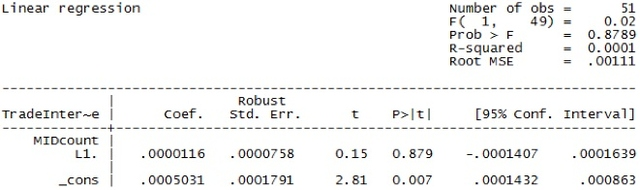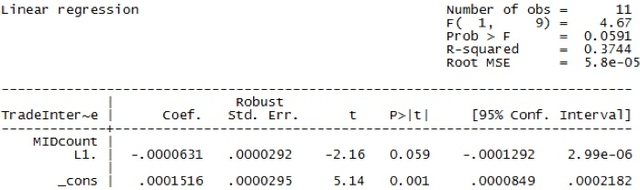# Trade and Conflict in South Asia: Examining the Relationship Between Trade Interdependence and Militarized Conflict

By
2012, Vol. 4 No. 08 | pg. 4/5 |

The India-Bangladesh dataset is the one with which I could not find a statistically significant relationship between trade interdependence and MID count. When I ran the dataset in SPSS as a simple bivariate linear regression and later in STATA as a time-lag linear regression, I found a slightly negative correlation between the two variables of interest that was not statistically significant at the 0.05 level or even at the 0.1 level. Therefore, of all the datasets, it appears as though India-Bangladesh is the only one that does not depict a strong correlation between the two variables- trade interdependence and MID occurrence. However, when I went back and looked at the dataset by hand, I noticed one particular time period of interest during which I could focus my research.

Overall, I think that the India-Bangladesh relationship could be explored further in order to determine if any other variables could help explain the level of trade and conflicts these two nations have engaged in over the past 30 years. However, this study will delve no deeper into this subject- this is a topic that can and should be addressed by future research.

India-Pakistan

The case study of India and Pakistan is an interesting one because the numerous linear regression tests I ran produced varying results. The original bivariate linear regression run in SPSS resulted in a slightly positive correlation between the variables of trade interdependence and MID occurrence, but it was not statistically significant at the 0.05 level. Moreover, the R2 value was 0.002, which means the best fit line approximates well for only 0.2 percent of the data points. Next, when I turned to STATA to run the time-lag linear regression with MID count from the previous year as the independent variable, the results showed a slightly positive, statistically insignificant correlation between the two variables of interest for the years 1950 to 2001 (see Table 1.7). Furthermore, the R2 value was 0.0001, which means the best fit line for this particular time-lag linear regression could only approximate well for 0.01 percent of the data points. Since I was unable to derive a conclusive relationship between the two variables of interest, I decided to then turn to breaking down the dataset into subsets for certain time ranges.

Table 1.7: India-Pakistan MID v. Trade Interdependence Time-Lag Linear Regression 1When I broke the dataset down into smaller portions, I found that every subset had a slightly negative correlation between the variables of MID count from the previous year and trade interdependence of the following year. While most of these results were statistically insignificant, I did find one time period that yielded a statistically significant, slightly negative relationship between the two variables of interest (see Table 1.8). The years from 1979 to 1989 are unique because during this time period, India and Pakistan were technically not engaged in a war (BBC Staff 2002), but they still had heightened tensions and MID occurrences. From my qualitative research, I found the two most likely causes of these tensions: between the years of 1981 and 1984, seven Indian Airlines flights to Pakistan had been hijacked and in 1985, an Air India flight was blown up by terrorists (Raman 2010) and in the late 1970s to late 1980s, both India and Pakistan reportedly began creating and testing nuclear weapons (Washington Post Staff 1998). Therefore, it is probable that the relationship between trade interdependence and MID occurrences between India and Pakistan from 1979 to 1989 was one that had MID occurrence from the previous year as the independent variable and trade interdependence the following year as the dependent variable. As India and Pakistan’s tensions escalated in the late 1970s to late 1980s, trade interdependence between the two nations went down: a theory that is supported by the negative, statistically significant results of my time-lag regression.

Table 1.8: India-Pakistan MID v. Trade Interdependence Time-Lag Linear Regression 2India-Sri Lanka

The last dyad pair I investigated for my case studies is India and Sri Lanka. When the bivariate linear regression I ran for this country pair using trade interdependence as the independent variable and MID count as the dependent variable resulted in a moderately negative, statistically insignificant correlation, I decided to run the time-lag regression next. When I ran the time-lag regression in STATA for the entire time range of 1950 to 2001, the results showed a slightly negative relationship between the two variables of interest that had a p-value of nearly zero (see Table 1.9). The fact that the p-value was rounded to zero is important because the closer the p-value is to zero, the more statistically significant the results are. Therefore, the relationship I found between the variables MID count from the previous year and trade interdependence from the following year is one that definitely exists. However, the R2 value for the time range of 1950 to 2001 was only 0.0661, which means only 6.61 percent of the data points can be approximated well by the regression best-fit line.

Table 1.9: India-Sri Lanka Trade Interdependence v. MID Count Time-Lag Linear Regression 1As a result, I decided to narrow down the time range to approximately a decade and then look at the results again, especially focusing on the R2 value. When I ran the time-lag regression again, but this time for the years from 1982 to 1991, I found a slightly negative correlation between the two variables of interest yet again, but this time it was not statistically significant at the 0.05 level. However, the best-fit line for this regression could approximate well for 20.7 percent of the data points, so it was useful in running this regression to see how the results changed (see Table 1.10). One reason why the India-Sri Lanka dataset may not be as helpful in establishing a relationship between the variables of trade interdependence and MID occurrence is because the two countries only engaged in five MIDs in a nearly 50-year span. Therefore, while the results are interesting to look at and compare to the other country pairs, the correlations established by this country pair are difficult to generalize to the entire region of South Asia.

Table 1.10: India-Sri Lanka Trade Interdependence v. MID Count Time-Lag Linear Regression 2